# How To Do Calculation In VBA in Microsoft Excel 2010

In this article, you will learn how to perform calculation in Excel using VBA code.

Let us understand with an example:

We have some random numbers which we want to multiply & then add with some numbers for each cell.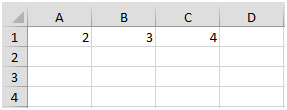If you have to perform calculation on the selected cells, then you need to follow the below steps:

Click on Developer tab

From Code group, select Visual Basic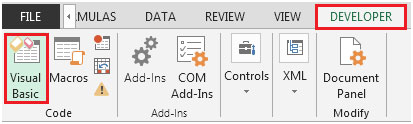Click on Insert, and then Module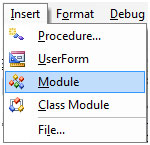This will create new module.

Enter the following code in the Module

Sub Calculation()

x = InputBox("Multiply by what?", Default:=1)

For Each cell In Selection

cell.Value = cell.Value * x + y

Next cell

End Sub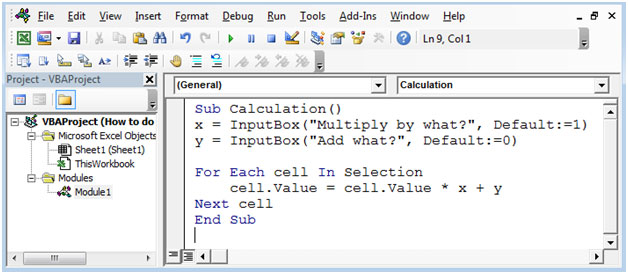Press ALT + F8 shortcut key for opening Macro window & then select the macro.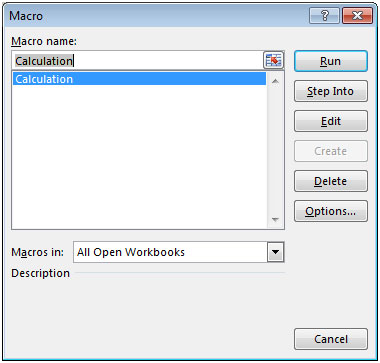Alternatively, you can press F5 to run the code in VBA screen.

After executing the macro, we will get the following input box to enter the number “Multiply by what”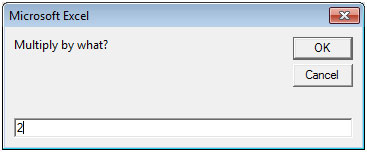After entering the value (2 in our example), the next input box will appear in front of you to enter the number to add (2 in our example). Refer below snapshot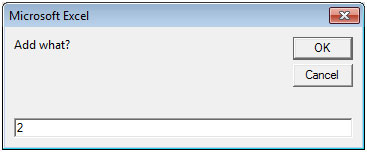We will get the output. Refer below snapshot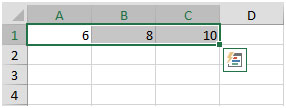In this way, we can make calculations according to the requirement of the user.

Terms and Conditions of use

The applications/code on this site are distributed as is and without warranties or liability. In no event shall the owner of the copyrights, or the authors of the applications/code be liable for any loss of profit, any problems or any damage resulting from the use or evaluation of the applications/code.